# Predict Blood Donations¶

The objective of this notebook is to predict if a blood donor will donate within a given time window, given parameters such as months since last donation (recency), number of donations made (frequency) , total volume of blood donated (cc) [monetary], months since first donation (time).

This is a warm-up problem in an ongoing competition at https://www.drivendata.org/competitions/2/warm-up-predict-blood-donations/page/7/

In [ ]:


In :
import pandas as pd
import numpy as np
import seaborn as sns
import matplotlib
import matplotlib.pyplot as plt

from sklearn.metrics import classification_report
from sklearn.metrics import confusion_matrix,log_loss
from sklearn.model_selection import cross_val_score

from imblearn import over_sampling as os
from imblearn import under_sampling as us
from imblearn import pipeline as pl
from imblearn.metrics import classification_report_imbalanced
from collections import Counter
from imblearn.combine import SMOTETomek
from sklearn.decomposition import PCA

from keras.models import Sequential
from keras.layers import Dense, Dropout
from keras.callbacks import EarlyStopping
from keras.utils import to_categorical

RANDOM_STATE = 2017

%matplotlib inline

In :
from jupyterthemes import jtplot

jtplot.style(ticks=True, grid=False)

In [ ]:


In [ ]:


In :
df = pd.read_csv("transfusion.data.txt")

In :
df.head()

Out:
Recency (months) Frequency (times) Monetary (c.c. blood) Time (months) whether he/she donated blood in March 2007
0 2 50 12500 98 1
1 0 13 3250 28 1
2 1 16 4000 35 1
3 2 20 5000 45 1
4 1 24 6000 77 0
In :
df.columns = ['months_since_last_donation', 'num_donations', 'vol_donations', 'months_since_first_donation', 'class']

In :
df.head()

Out:
months_since_last_donation num_donations vol_donations months_since_first_donation class
0 2 50 12500 98 1
1 0 13 3250 28 1
2 1 16 4000 35 1
3 2 20 5000 45 1
4 1 24 6000 77 0

class 1 => the donor donated blood in March 2007 [let's call them donors]
class 0 => the donor did not donate blood in March 2007 [ let's call them non-donors ]

In [ ]:



Number of instances of each class

In :
df['class'].value_counts()

Out:
0    570
1    178
Name: class, dtype: int64

we see that there is a class imbalance problem here. We can fix this issue by oversampling the minority class (1) or undersampling the majority class.

## EDA¶

Let's explore the dataset and find out if we can get some interesting insights.

In :
def ecdf(data):
"""
returns empirical CDF of a data
"""
n= len(data)
x = np.array(sorted(data))
y = np.arange(1,n+1)/(n*1.0)
return x,y


In [ ]:


In [ ]:


In :
plt.figure(figsize=(15,10))
_ = sns.kdeplot(df[df['class']==0]['months_since_last_donation'],cumulative=True,label='0')
_ = sns.kdeplot(df[df['class']==1]['months_since_last_donation'],cumulative=True,label='1')
_ = plt.xlabel('months since last donation')
_ = plt.ylabel('CDF')
_ = plt.title('CDF of recency of donors vs non-donors')
plt.show();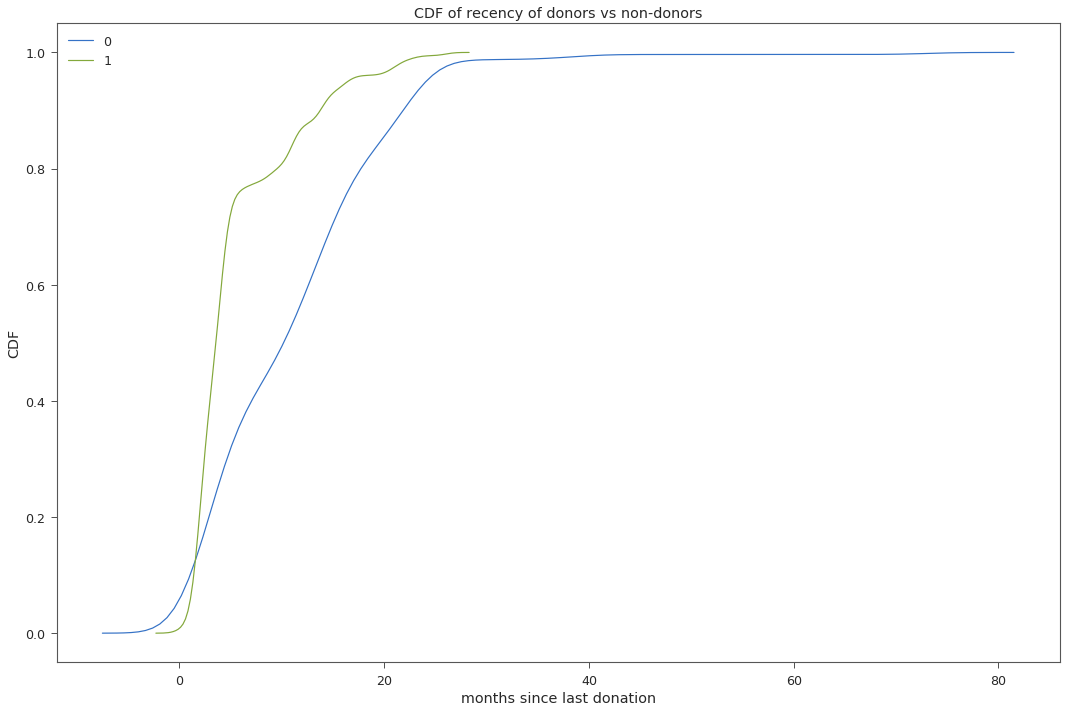People who are likely to donate blood again have donated blood more recently. The curve representing the CDF of donors (class 1) grows faster with less recency values than CDF of non-donors (class 0).

In :
plt.figure(figsize=(15,10))
_ = sns.kdeplot(df[df['class']==0]['num_donations'],cumulative=True,label='0')
_ = sns.kdeplot(df[df['class']==1]['num_donations'],cumulative=True,label='1')
_ = plt.xlabel('number of donations')
_ = plt.ylabel('CDF')
_ = plt.title('CDF of frequency of donors vs non-donors')
plt.show()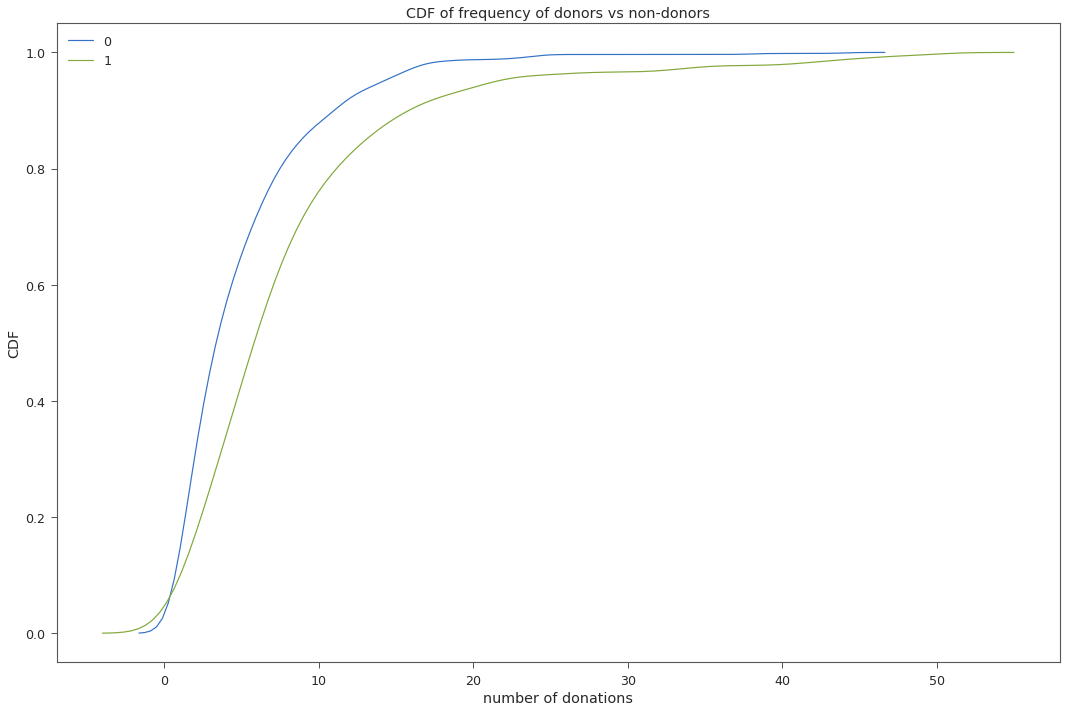From the above plot, we observe that donors donate more frequently than non-donors

In :
plt.figure(figsize=(15,10))
_ = sns.kdeplot(df[df['class']==0]['vol_donations'],cumulative=True,label='0')
_ = sns.kdeplot(df[df['class']==1]['vol_donations'],cumulative=True,label='1')
_ = plt.xlabel('volume of blood donated')
_ = plt.ylabel('CDF')
_ = plt.title('CDF of monetary value of donors vs non-donors')
plt.show()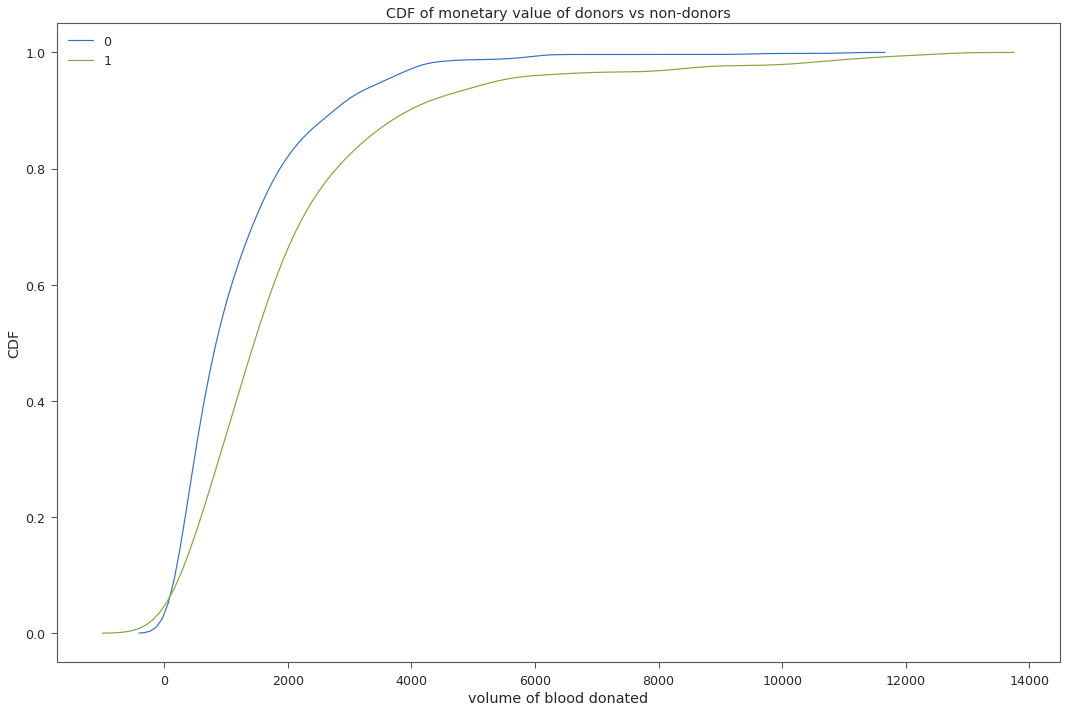The above CDF looks similar to the previous one. Frequency and monetary values are highly correlated. Here again, donars donate more volumne of blood than non-donors

In :
plt.figure(figsize=(15,10));
_ = sns.lmplot(x='num_donations',
y='vol_donations',
hue='class',
fit_reg=False,
data=df);
_ = plt.title("Correlation between frequency and monetary");
plt.show();

<matplotlib.figure.Figure at 0x7f40856a6990>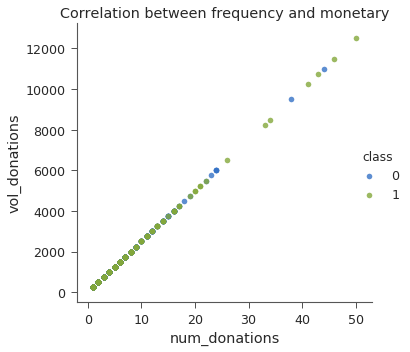In [ ]:


In :
plt.figure(figsize=(15,10))
_ = sns.kdeplot(df[df['class']==0]['months_since_first_donation'],cumulative=True,label='0')
_ = sns.kdeplot(df[df['class']==1]['months_since_first_donation'],cumulative=True,label='1')
_ = plt.xlabel('months since first donation')
_ = plt.ylabel('CDF')
_ = plt.title('CDF of months since first donation of donors vs non-donors')
plt.show()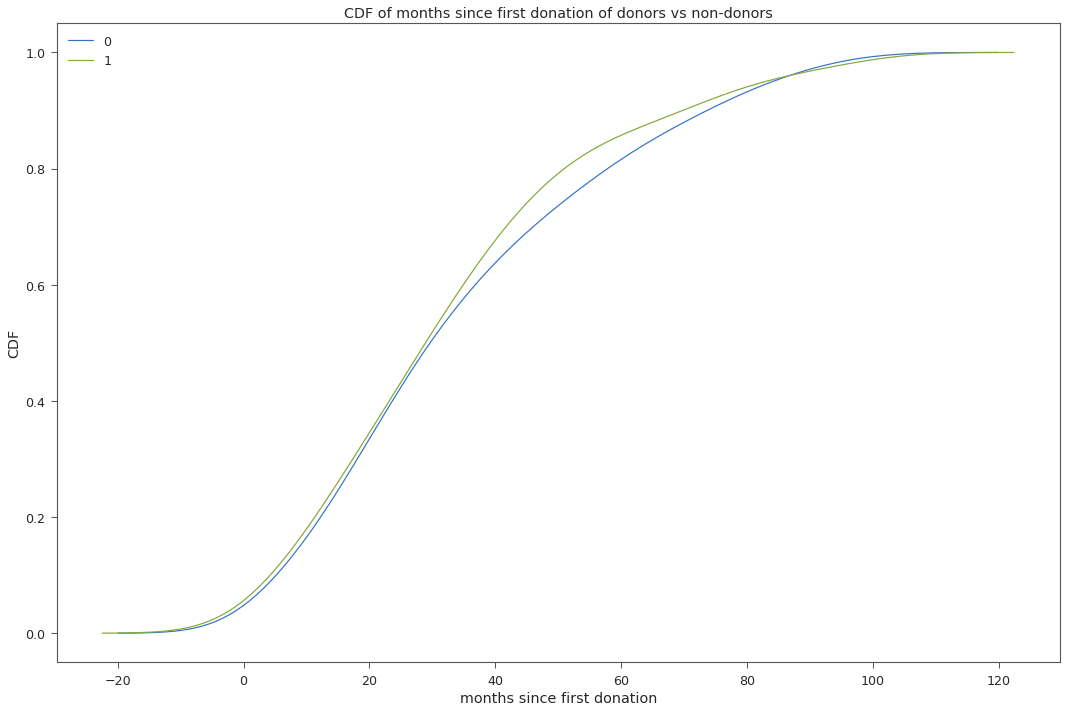CDF of months since first donations is not so differentiable between the two classes.

In :
df_long = pd.melt(df,id_vars='class',var_name='feature')
plt.figure(figsize=(15,10))
_ = sns.boxplot(x='feature',y='value',hue='class',data=df_long[df_long.feature!='vol_donations'])
plt.margins(0.02)
_ = plt.title('Boxplot of recency,frequency and time')
plt.show()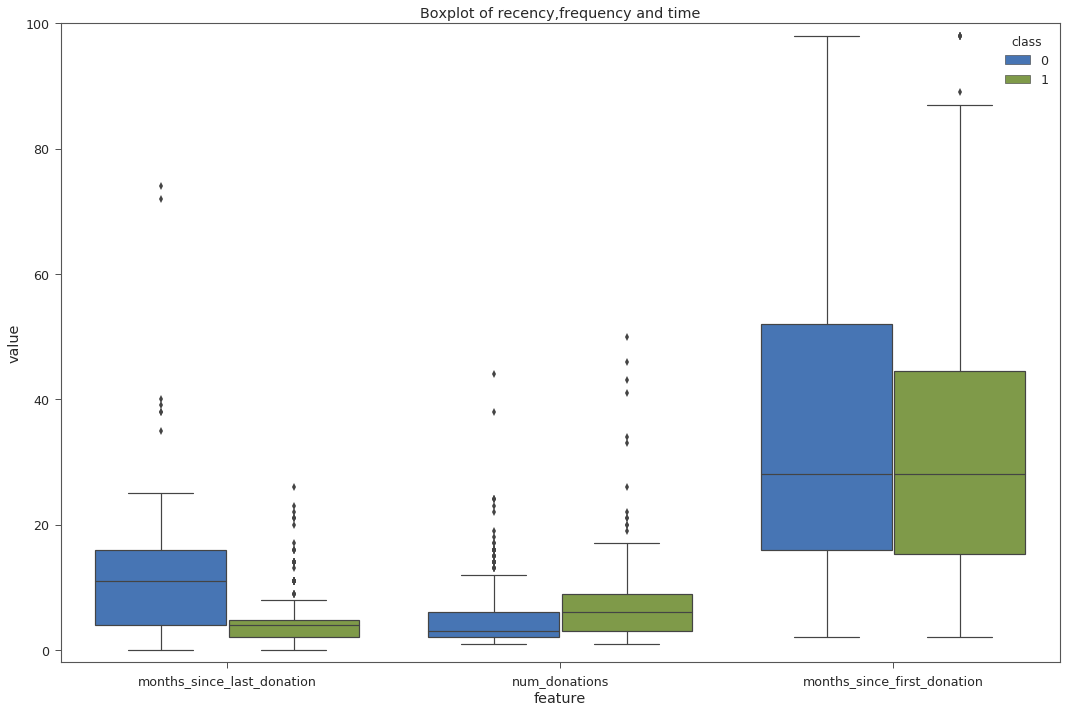we see that there are few outliers in recency and frequency for each of the classes. Should we remove them? What's the story behind these outliers?

In [ ]:


In [ ]:


In [ ]:



## Prepare Data¶

In :
from sklearn.model_selection import train_test_split


Let's split our dataset into training and testing. We'll drop 'monetary' feature from our training data since it has high correlation to 'frequency'. It is a good practise to standardise our data.

In :
x_train, x_test, y_train,y_test = train_test_split(df.drop(['class','vol_donations'],axis=1), df['class'], test_size = 0.1, random_state=2017)

In :
from sklearn.preprocessing import StandardScaler
scaler = StandardScaler()
x_train_scaled = scaler.fit_transform(x_train)
x_test_scaled = scaler.transform(x_test)

In :
x_train_scaled.mean(),x_train_scaled.std()

Out:
(-8.7982012847956928e-19, 1.0)
In :
x_test_scaled.mean(),x_test_scaled.std()

Out:
(0.058943449584060933, 1.155008299202017)
In [ ]:


In [ ]:



## Resampling and Visualizations¶

In :
# Instanciate a PCA object for the sake of easy visualisation
pca = PCA(n_components=2,random_state=RANDOM_STATE)
# Fit and transform x to visualise inside a 2D feature space
X_vis = pca.fit_transform(x_train_scaled)

pca_original_df = pd.DataFrame(X_vis,y_train).reset_index()
pca_original_df.columns = ['class','x','y']

# Apply SMOTE + Tomek links
sm = SMOTETomek()
X_resampled, y_resampled = sm.fit_sample(x_train_scaled, y_train)
X_res_vis = pca.transform(X_resampled)

pca_resampled_df = pd.DataFrame(X_res_vis,y_resampled).reset_index()
pca_resampled_df.columns = ['class','x','y']


Visualizing original data in 2D

In :
_ = sns.lmplot(x='x',
y='y',
hue='class',
x_jitter=True,
y_jitter=True,
fit_reg=False,
size=7,
aspect = 1.5,
data=pca_original_df,
scatter_kws={'alpha':0.5})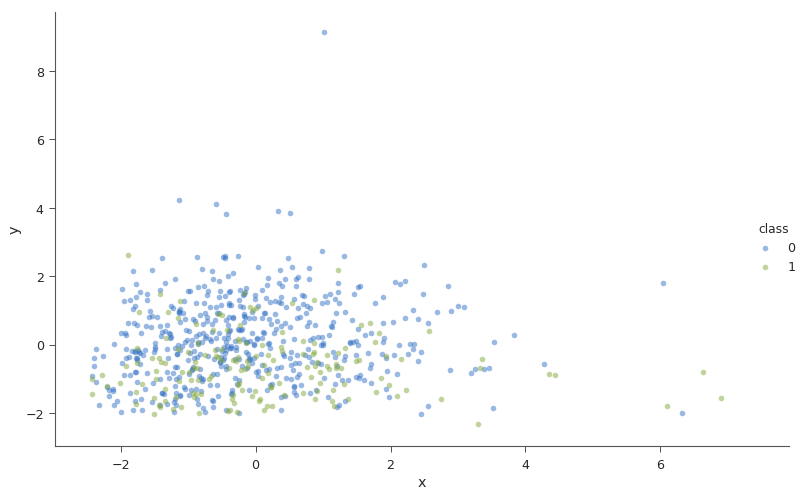Visualizing resampled data in 2D

In :
_ = sns.lmplot(x='x',
y='y',
hue='class',
x_jitter=True,
y_jitter=True,
fit_reg=False,
size=7,
aspect = 1.5,
data=pca_resampled_df,
scatter_kws={'alpha':0.5})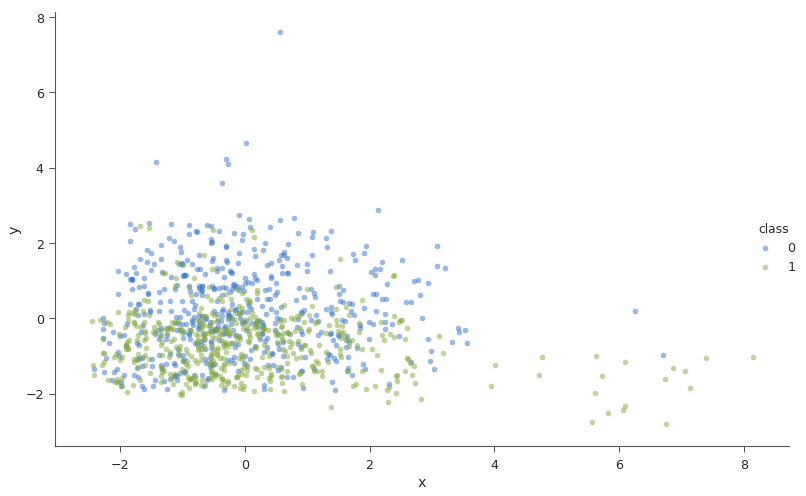In [ ]:



I have tried building models on the resampled dataset, but the performance did not improve. Let's try doing some feature engineering on the dataset.

In [ ]:


In :
df.head()

Out:
months_since_last_donation num_donations vol_donations months_since_first_donation class
0 2 50 12500 98 1
1 0 13 3250 28 1
2 1 16 4000 35 1
3 2 20 5000 45 1
4 1 24 6000 77 0

submission

In :
def create_submission(clf):
test.columns = ['id','months_since_last_donation','num_donations','vol_donations','months_since_first_donation']
submit_id, submit_test = test['id'] , test.drop(['id','vol_donations'],axis=1)
submit_test_scaled = scaler.transform(submit_test) #scale the data
predictions = clf.predict_proba(submit_test_scaled)
predictions = predictions[:,1] #only predictions for class-1 needs to be submitted
pred_report = pd.DataFrame(predictions.tolist(),index=submit_id,columns=["Made Donation in March 2007"])
return pred_report

In [ ]:



## Utility Functions¶

In :
import itertools

def plot_confusion_matrix(cm, classes,
normalize=False,
title='Confusion matrix',
cmap=plt.cm.Accent):
"""
This function prints and plots the confusion matrix.
Normalization can be applied by setting normalize=True.
"""
plt.figure(figsize=(10,7))
plt.imshow(cm, interpolation='nearest', cmap=cmap)
plt.title(title)
plt.colorbar()
tick_marks = np.arange(len(classes))
plt.xticks(tick_marks, classes, rotation=45)
plt.yticks(tick_marks, classes)

if normalize:
cm = cm.astype('float') / cm.sum(axis=1)[:, np.newaxis]
print("Normalized confusion matrix")
else:
print('Confusion matrix')

print(cm)

thresh = cm.max() / 2.
for i, j in itertools.product(range(cm.shape), range(cm.shape)):
plt.text(j, i, cm[i, j],
horizontalalignment="center",
color="white" if cm[i, j] > thresh else "black")

plt.tight_layout()
plt.ylabel('True label')
plt.xlabel('Predicted label')
plt.show()
return

def prediction_report(true_label,predicted_label,classes=[0,1]):

report = classification_report(true_label, predicted_label)
print "classification report:\n",report
cnf_matrix = confusion_matrix(true_label, predicted_label)
np.set_printoptions(precision=2)
# Plot non-normalized confusion matrix
plt.figure(figsize=(15,10))
plot_confusion_matrix(cnf_matrix, classes=classes,title='Confusion matrix, without normalization')
return

In [ ]:


In [ ]:



## Models¶

In :
from sklearn.svm import SVC;
from sklearn.linear_model import LogisticRegression
from sklearn.ensemble import RandomForestClassifier
from xgboost import XGBClassifier;
from keras.wrappers.scikit_learn import KerasClassifier
np.random.seed(2017)

early_stopping_monitor = EarlyStopping(monitor='val_loss',patience=5)

def create_mlp():
model = Sequential()
return model

svc = SVC(verbose=1,C=10,gamma=0.1,kernel='rbf',random_state=2017,probability=True);
svc.fit(x_train_scaled,y_train);

logit_model = LogisticRegression(random_state=2017)
logit_model.fit(x_train_scaled,y_train)

rf = RandomForestClassifier(n_estimators=100,max_depth=6,class_weight='balanced',n_jobs=4,random_state=2017)
rf.fit(x_train_scaled,y_train)

xgb = XGBClassifier(learning_rate=0.03,max_depth=5,n_estimators=250,reg_alpha=0.01)
xgb.fit(x_train_scaled,y_train)

mlp = KerasClassifier(build_fn=create_mlp, epochs=20, batch_size=10, validation_split=0.2,verbose=False)

models = {'SVC': svc,
'Logistic Regression': logit_model,
'Random Forest': rf,
'XGBoost': xgb,
'mlp': mlp
}

[LibSVM]

Grid search for the best parameters of our models

In :
from sklearn.model_selection import GridSearchCV

parameters = {'kernel':('linear', 'rbf'), 'C':[1, 10,100,1000], 'gamma': [1e-1,1e-2,1e-3,1,10,100]}
svc_grid = SVC(probability=True,verbose=True)
clf = GridSearchCV(svc_grid, parameters,scoring='neg_log_loss',verbose=True,n_jobs=4)
clf.fit(x_train_scaled,y_train)

Fitting 3 folds for each of 48 candidates, totalling 144 fits

[Parallel(n_jobs=4)]: Done 128 tasks      | elapsed:  6.0min

[LibSVM]
[Parallel(n_jobs=4)]: Done 144 out of 144 | elapsed: 10.1min finished

Out:
GridSearchCV(cv=None, error_score='raise',
estimator=SVC(C=1.0, cache_size=200, class_weight=None, coef0=0.0,
decision_function_shape=None, degree=3, gamma='auto', kernel='rbf',
max_iter=-1, probability=True, random_state=None, shrinking=True,
tol=0.001, verbose=True),
fit_params={}, iid=True, n_jobs=4,
param_grid={'kernel': ('linear', 'rbf'), 'C': [1, 10, 100, 1000], 'gamma': [0.1, 0.01, 0.001, 1, 10, 100]},
pre_dispatch='2*n_jobs', refit=True, return_train_score=True,
scoring='neg_log_loss', verbose=True)
In :
pd.DataFrame(clf.cv_results_)[['mean_test_score',
'mean_train_score',
'param_C',
'param_gamma',
'param_kernel',
'rank_test_score']].sort_values('rank_test_score')[:5]

Out:
mean_test_score mean_train_score param_C param_gamma param_kernel rank_test_score
13 -0.489120 -0.470714 10 0.1 rbf 1
7 -0.494660 -0.456200 1 1 rbf 2
39 -0.496060 -0.474736 1000 0.01 rbf 3
25 -0.497201 -0.459765 100 0.1 rbf 4
1 -0.505502 -0.490678 1 0.1 rbf 5
In [ ]:



Cross-validation

In :
np.random.seed(2017)
print "Cross-validation score"
print  "========================="
for model_name, model in models.items():
if model_name=='mlp':
cv_score = cross_val_score(estimator=model,X=x_train_scaled,y=to_categorical(y_train),
scoring='neg_log_loss',
cv=10,
n_jobs=4,
verbose=False,
fit_params={'callbacks':[early_stopping_monitor]})
else:
cv_score = cross_val_score(estimator=model,
X=x_train_scaled,
y=y_train,
cv=10,
n_jobs=4,
scoring='neg_log_loss',
verbose=False)
print model_name," ",-cv_score.mean(),"+/-",cv_score.std()

Cross-validation score
=========================
mlp   0.478453494027 +/- 0.0807720022143
XGBoost   0.535469900327 +/- 0.100141966183
SVC   0.491209526548 +/- 0.0481133206892
Logistic Regression   0.47551648798 +/- 0.0601495385611
Random Forest   0.556231217696 +/- 0.0740108456588

In [ ]:



Test the models on test set

In :
np.random.seed(2017)
print "Log loss"
print "=============="
for model_name,model in models.items():
if model_name == 'mlp':
mlp.fit(x_train_scaled,to_categorical(y_train)) ##need to fit the model before predicting
y_pred = model.predict_proba(x_test_scaled)
print model_name,": ",log_loss(y_test,y_pred)

Log loss
==============
mlp :  0.519841400829
XGBoost :  0.592722008402
SVC :  0.516236741052
Logistic Regression :  0.52176733679
Random Forest :  0.612271296484

In [ ]:



## Feature Engineering¶

In :
df.head()

Out:
months_since_last_donation num_donations vol_donations months_since_first_donation class
0 2 50 12500 98 1
1 0 13 3250 28 1
2 1 16 4000 35 1
3 2 20 5000 45 1
4 1 24 6000 77 0
In :
df['num_donations_per_month'] = df['num_donations']*1.0/df['months_since_first_donation']

In :
df['months_between_first_n_last_donation'] = df['months_since_first_donation'] - df['months_since_last_donation']

In :
df.head()

Out:
months_since_last_donation num_donations vol_donations months_since_first_donation class num_donations_per_month months_between_first_n_last_donation
0 2 50 12500 98 1 0.510204 96
1 0 13 3250 28 1 0.464286 28
2 1 16 4000 35 1 0.457143 34
3 2 20 5000 45 1 0.444444 43
4 1 24 6000 77 0 0.311688 76
In :
np.corrcoef(df.months_since_first_donation,df.months_between_first_n_last_donation)

Out:
array([[ 1.  ,  0.94],
[ 0.94,  1.  ]])
In [ ]:


In :
corr = df.drop('class',axis=1).corr()
plt.figure(figsize=(15,10))
sns.heatmap(corr,
xticklabels=corr.columns.values,
yticklabels=corr.columns.values,
annot=True
);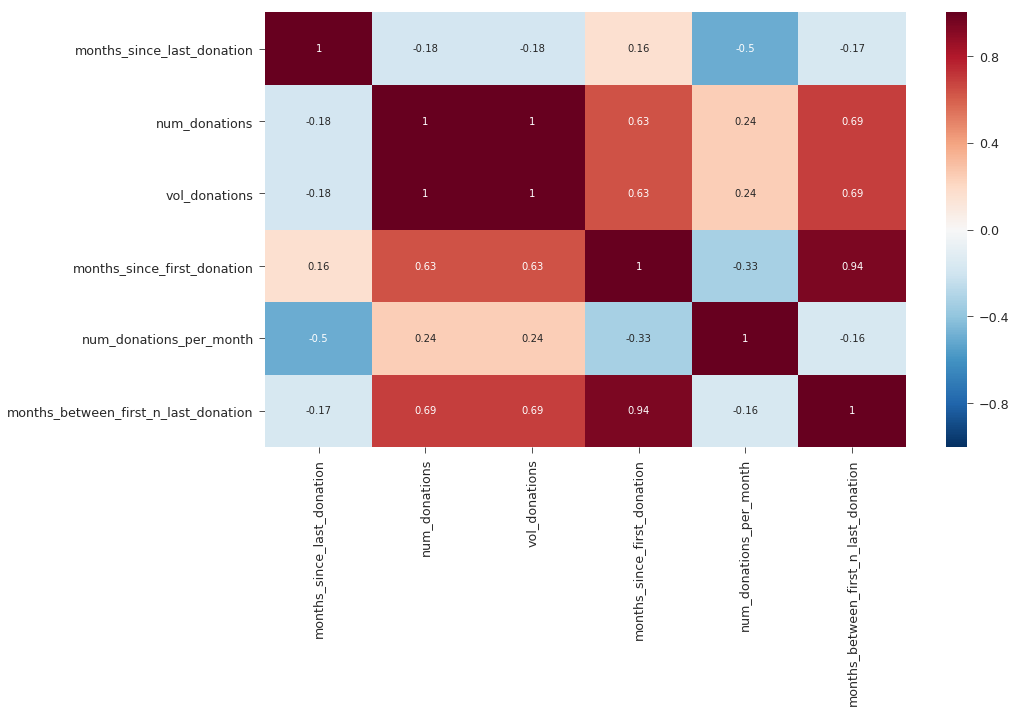In [ ]: